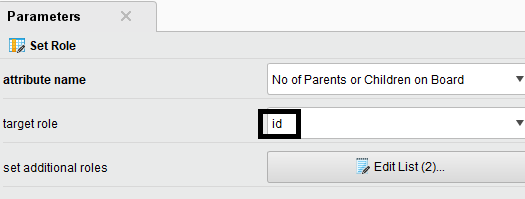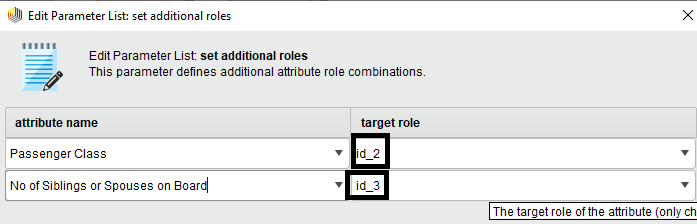# How to use cluster based on specific attributes

Member Posts: 2Newbie
edited May 2020 in Help
Greetings, everyone

I'm trying to use k-means to cluster and i'm only interested in a couple of attributes to do my clustering with. Problem is, i don't want to use select attributes because i don't want to get rid of the attributes i'm not interested in clustering with because I'll be needing them for illustrative purposes and for later use. Can someone clarify how to use k-means to cluster data based on specific attributes or features while also keeping other features in the dataset that won't be used for clustering. thanks.
Tagged:

• Moderator, RapidMiner Certified Analyst, Member Posts: 1,195Unicorn
Solution Accepted
Hi @omark,

You can use the Set Role operator and set a special role to the attribute(s) you don't need, for example id, id_2,id_3 etc. . This way, the
attributes with the special roles are not taken into account  for the clustering.Regards,

Lionel

• Member Posts: 2Newbie
also here's the xml code i ended up with i want to use the attributes total day charge, total eve charge, total night charge and total intel charge in my clustering model while not getting rid of the other attributes in the dataset that won't be used for clustering
<?xml version="1.0" encoding="UTF-8"?><process version="9.5.000">
<context>
<input/>
<output/>
<macros/>
</context>
<operator activated="true" class="process" compatibility="9.4.000" expanded="true" name="Process">
<parameter key="logverbosity" value="init"/>
<parameter key="random_seed" value="2001"/>
<parameter key="send_mail" value="never"/>
<parameter key="process_duration_for_mail" value="30"/>
<parameter key="encoding" value="SYSTEM"/>
<process expanded="true">
<operator activated="true" class="retrieve" compatibility="9.5.000" expanded="true" height="68" name="Retrieve train" width="90" x="45" y="85">
<parameter key="repository_entry" value="//Local Repository/train"/>
</operator>
<operator activated="true" class="split_data" compatibility="9.5.000" expanded="true" height="103" name="Split Data" width="90" x="179" y="85">
<enumeration key="partitions">
<parameter key="ratio" value="0.7"/>
<parameter key="ratio" value="0.3"/>
</enumeration>
<parameter key="sampling_type" value="automatic"/>
<parameter key="use_local_random_seed" value="false"/>
<parameter key="local_random_seed" value="1992"/>
</operator>
<operator activated="true" class="select_attributes" compatibility="9.5.000" expanded="true" height="82" name="Select Attributes" width="90" x="313" y="85">
<parameter key="attribute_filter_type" value="subset"/>
<parameter key="attribute" value=""/>
<parameter key="attributes" value="Total day charge|Total eve charge|Total intl charge|Total night charge"/>
<parameter key="use_except_expression" value="false"/>
<parameter key="value_type" value="attribute_value"/>
<parameter key="use_value_type_exception" value="false"/>
<parameter key="except_value_type" value="time"/>
<parameter key="block_type" value="attribute_block"/>
<parameter key="use_block_type_exception" value="false"/>
<parameter key="except_block_type" value="value_matrix_row_start"/>
<parameter key="invert_selection" value="false"/>
<parameter key="include_special_attributes" value="false"/>
</operator>
<operator activated="true" class="concurrency:k_means" compatibility="9.5.000" expanded="true" height="82" name="Clustering" width="90" x="447" y="85">
<parameter key="remove_unlabeled" value="false"/>
<parameter key="k" value="5"/>
<parameter key="max_runs" value="10"/>
<parameter key="determine_good_start_values" value="true"/>
<parameter key="measure_types" value="BregmanDivergences"/>
<parameter key="mixed_measure" value="MixedEuclideanDistance"/>
<parameter key="nominal_measure" value="NominalDistance"/>
<parameter key="numerical_measure" value="EuclideanDistance"/>
<parameter key="divergence" value="SquaredEuclideanDistance"/>
<parameter key="kernel_gamma" value="1.0"/>
<parameter key="kernel_sigma1" value="1.0"/>
<parameter key="kernel_sigma2" value="0.0"/>
<parameter key="kernel_sigma3" value="2.0"/>
<parameter key="kernel_degree" value="3.0"/>
<parameter key="kernel_shift" value="1.0"/>
<parameter key="kernel_a" value="1.0"/>
<parameter key="kernel_b" value="0.0"/>
<parameter key="max_optimization_steps" value="100"/>
<parameter key="use_local_random_seed" value="false"/>
<parameter key="local_random_seed" value="1992"/>
</operator>
<operator activated="true" class="apply_model" compatibility="9.5.000" expanded="true" height="82" name="Apply Model" width="90" x="447" y="187">
<list key="application_parameters"/>
<parameter key="create_view" value="false"/>
</operator>
<connect from_op="Retrieve train" from_port="output" to_op="Split Data" to_port="example set"/>
<connect from_op="Split Data" from_port="partition 1" to_op="Select Attributes" to_port="example set input"/>
<connect from_op="Split Data" from_port="partition 2" to_op="Apply Model" to_port="unlabelled data"/>
<connect from_op="Select Attributes" from_port="example set output" to_op="Clustering" to_port="example set"/>
<connect from_op="Clustering" from_port="cluster model" to_op="Apply Model" to_port="model"/>
<connect from_op="Apply Model" from_port="labelled data" to_port="result 1"/>
<portSpacing port="source_input 1" spacing="0"/>
<portSpacing port="sink_result 1" spacing="0"/>
<portSpacing port="sink_result 2" spacing="0"/>
</process>
</operator>
</process>